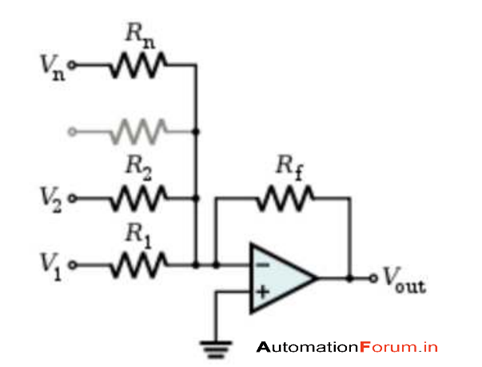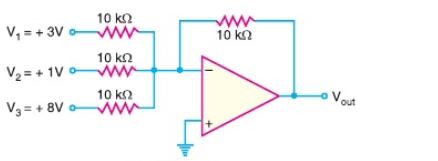# SUMMING AMPLIFIER AND ITS APPLICATIONS

SUMMING AMPLIFIER AND ITS APPLICATIONS

Summing amplifier is an inverted Op-amp which can accept two or more inputs it is used to combine the voltages present in two or more inputs to single output voltage it is also called as summing inverter or voltage adder by adding more input resistors to the input which have equal value to the original input resistor and thus we can create a summing amplifier. Summing amplifier’s output voltage is proportional to the negative of the algebraic sum of its input voltages. It is also named as adder which is used to add two signal voltages it is really easy to construct the circuit of this summing amplifier and it helps to add many signals together it is widely used for many electronics based circuits offset error of the operational amplifier could be rectified by this audio mixer is another example for this it will add many waveforms from different channels and then it will send it to the recorder we could also change the input or gain without creating any problems in the input section ( the summing amplifier circuit changes the input signal )Summing amplifier adds many voltages Vout = - Rf (V1/R1+V2/R2………Vn/Rn) R1=R2= …………= Rn Rf is independent Vout = - Rf/R1 ( V1 + V2 + ……….Vn) When R1=R2= ………Rn=Rf Vout = -( V1+V2………Vn)

Summing amplifierAll the three input resistor values are equal and it is equal to the value of the feedback resistor and thus the gain of the summing amplifier is 1 The output voltage is the algebraic sum of three input voltages

VOUT = -(V1+V2+V3) =-(3+1+8)= -12v

APPLICATIONS OF SUMMING AMPLIFIERS

• It can be used to add the signals
• It is used in an audio mixer to add different signals with equal gains
• Many resistors are used in the input of the summing amplifier it could be used to change a binary number to a voltage in an AC
• It can be used to apply a Dc offset voltage with an AC signal voltage
• It can be used as an averaging amplifier with the proper input and feedback resistor values a summing amplifier can be modified to provide an output voltage which is equal to the average input values. Summing amplifiers will act as an averaging amplifier when two conditions are met those are (1) All input resistors are equal in value (2) Ratio to the feedback resistor from any input resistor is equal to the number of input circuits
• A summing amplifier can act as a subtractor by providing an output voltage which is equal to the difference of two voltages
• summing amplifier can be used as a digital to analog converter